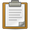This is a documentation subpage, where instructions, categories, or other information are stored for technical reasons. To view the Template page itself, see Template:Valid.

## Purpose

Determines whether something is valid in a certain context. Currently only implemented for determining if a number is within the precision that Wikipedia expressions can handle.

## Returns

true if the argument is valid, false if it is not.

## Examples

`{{valid|number=A}}` = false (not a number)
`{{valid|number=1234}}` = true
`{{valid|number=+1234}}` = true
`{{valid|number=-1234}}` = true
`{{valid|number=(1234)}}` = true (one pair of parenthesis is allowed)
`{{valid|number=--1234}}` = false (incorrect sign use)
`{{valid|number=1234567890}}` = true
`{{valid|number=12345678901234567890}}` = false (too large)
`{{valid|number=1.234567890}}` = true
`{{valid|number=1.2345678901234567890}}` = false (too many decimals)

## Performance impact

Template:Valid returns "true" for a valid, single number (2+2 gives "false"), and allows scientific notation (such as: -3.45E-07). The precision limit is determined live, for whichever server is formatting the page, typically allowing 14-digit precision (plus trailing zeroes), but it does not detect extreme precision problems dropping minor end-digits:

• cannot reject: -10020030040050000000000000000.70, treated as -1.002003004005E+28

The template has been written with minimal markup text, and could be used 50,000-13,000 times per page, or less when all 30-digit numbers. Template:Valid is typically used at upper levels of other templates, so it is unlikely to trigger expansion-depth problems. However, it has an expansion-depth of 8 levels, and returns "false" if used when nested too deep inside other templates, such as at level 33 when the expansion depth limit is 40.